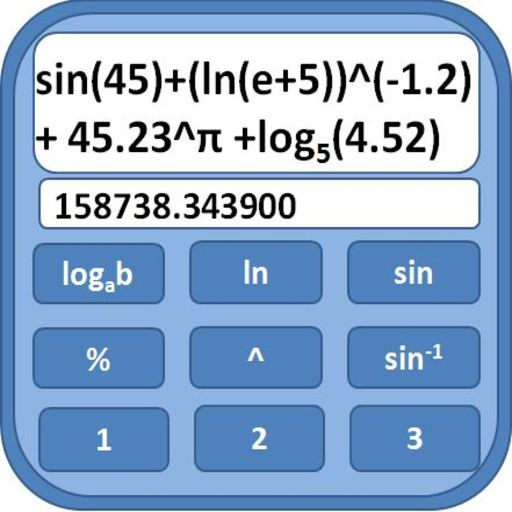# Voting Now

##### Creativity
×## Scientific Calculator

### by Wolyapps

Free
Design
Usability
Creativity
Average
16 USERS VOTED
##### TAGS
###### Description

Features:
1) Easy to edit large expressions. Just click anywhere on clipboard and edit your expression.
2) It can also show number as big as factorial of 5000. Just scroll down to see such big numbers.
3) No need to press shift. Just scroll down to use more functions.
4) You can write equations with 500 operands.
5) You can use Infinite Parentheses
Functions:
1) Basic Math Operations such as divide,add,subtract,multiply,modulo,factorial
2) Can calculate nth root of a number
3) Can calculate factorial up to 5000
4) Can Evaluate log with base between 1-100
5) Trigonometric Functions
6) Number Base Conversion from 2 to 16
7) Matrix operations
8) You can perform operations on large numbers

Note :
ln(23)^2 will be evaluated as ln(23^2) i.e. first 23^2 will evaluated which is 529 then ln(529) will be evaluated. If you want ln(23) should get evaluated first then write (ln(23))^2. Please use parentheses to get proper result otherwise you will get unexpected result.

View All